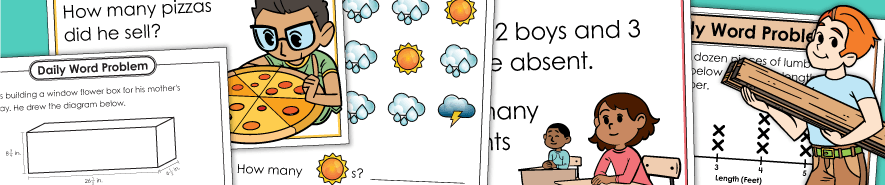# Daily Word Problems## Daily Word Problems

Kindergarten (Level K)

In Kindergarten, daily math word problem series will allow children to practice counting numbers to 20, comparing numbers (using more, most, fewest, etc.), adding and subtracting within 10, and identifying basic shapes.

Daily word problem worksheets for 1st grade students. Most problems require basic addition or subtraction. There are also occasional problems related to counting money and skip counting.

This page has 2nd grade daily math word problems. At this level, we have both single-step and multi-step problems. Single, double, and triple-digit addition and subtraction are frequently featured. Other skills include elapsed time, counting money, and repeated addition (multiplication).

In third grade, students will be presented with 2, 3, and 4-digit addition and subtraction problems, as well as questions involving basic multiplication, basic division, place value, counting money, tables & charts, and fractions.

In 4th grade, the daily word problems will consist of more multi-step math, covering operations with whole numbers, fractions, and decimals. There will also be questions related to place value, angles, graphs, and measurement conversions.

These 5th grade daily math worksheets continue to build on elementary math skills. Multi-step problems cover operations with whole numbers, as well as fractions and decimals. Understanding place value, calculating measurements, and reading charts and graphs are among the other skills covered in these worksheets.

Math Buzz

This is a different set of daily review worksheets, featuring a variety of math skills. Each day, students have 5 math questions to answer. Not all problems are word problems in this set.